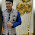## Monday, 20 October 2014

### CBSE Class 9 - Science - Gist on Work, Energy and Power

₩øяк €ηεґℊ⑂ @ηⅾ ℙ☺ωℯґ

Study Points

1. Work is said to be done if a force is applied on the object and it is displaced from its original position.

2. Three things are necessary for the performance of work:
• There must be an applied force F.
• There must be a displacement x.
• The force must have a component along the displacement
3. The work done W by a force F on an object is
W = Fd cos θ
where d is the displacement of an object.

θ is the angle between the force and the displacement.

4
. If displacement is along the direction of work i.e. θ = 0°
W = Fd

5. If displacement is opposite to the force i.e. θ = 180°
W = -Fd

6. if the displacement is perpendicular to the force (i.e. θ = 90°), then work done is zero.

7. Work done on an object by a force would be zero if the displacement of the object is zero.

8. Work is a scalar quantity.

9. Unit of work is Joule (J).

10.  1 Joule = 1 newton × 1 metre = 1 Nm
11. One Joule of work is to be done on an object when a force of one newton displaces it by one metre along the time of action of the force.

12. In the Force-displacement graph, the area enclosed by the force-displacement curve represents the work done by a variable force.

13. The total capacity of doing work by a body is called its energy.

14. Energy is a scalar quantity.

15. Unit of energy is Joule (J).

16. Kinetic Energy(KE): The energy possessed by a body by virtue of its motion is called kinetic energy.

17. KE = (½).m.v2

18. Potential Enegry (PE): The energy stored in a body by virtue of its position of state is called potential energy.

19. Surface of earth is usually considered as reference of zero potential energy.

20. Gravitational Potentional Energy = m.g.h
21. A stretched or compressed spring has elastic potential energy.

22. Mechanical Energy is the sum of  kinetic energy and potential energy.

23. Relationship between work and energy: Work done by external forces on a system is equal to the increase in its energy.

24. Relationship between K.E. and Momentum:
E = (½).m.v2 =  m2.v2/2m = p2/2m
⇒ E = √2mE

25Law of Conservation of Energy:  Energy can neither be created nor be destroyed. It can only be transformed from one form to another.

26. The total energy of the universe remains constant.

27. Power: The rate of doing work by a body is called its power.

28. Unit of power is Watt (W) i.e. 1 W = 1J/1s

29. Power = Work/time

30. 1 Horse Power (HP) = 746 Watt

31. Commercial Unit of Energy is kilowatt hour (kwh)

32. 1 kWh = 1000 W × 1 hour = 1000 × 3600s = 3.6 × 106J#### 1 comment:

1.how can i copy it plz..............